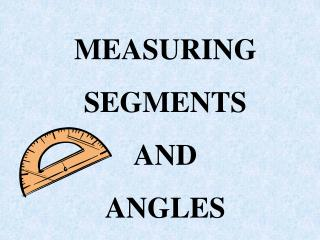DownloadDownload PresentationMEASURING SEGMENTS AND ANGLES

# MEASURING SEGMENTS AND ANGLES

Télécharger la présentation## MEASURING SEGMENTS AND ANGLES

- - - - - - - - - - - - - - - - - - - - - - - - - - - E N D - - - - - - - - - - - - - - - - - - - - - - - - - - -
##### Presentation Transcript

1. MEASURING SEGMENTS AND ANGLES

2. Assignment Page 29 - 30 2 – 30 even 31, 32, 34, 36, 42, 44, 46, 70, 72, 76, 78

3. Ruler Postulate 1- 5 The distance between any two points is the absolute value of the difference of the corresponding numbers Example: Length of AB is a – b which in this Case would be 2 – 5 Or the - 3 which is 3 B A

4. Congruent segments segments of the same length A B C D AB = CD or AB = CD The two tick marks is a way of showing that the two segments are congruent

5. A B C D E Compare CD and DE CD = -2 – 0 = -2 = 2 DE = 0 – 2 = - 2 = 2 CD = DE

6. Segment Addition Postulate 1- 6 If three points A, B, and C are collinear and B is between A and C, then AB + BC = AC Example : From previous CD = 2 and DE = 2 2 + 2 = 4 CE = -2 -2 = -4 = 4 A B C D E

7. 4x – 202x + 30 E F G EG = 100. Find the value of x, then EF and FG EF + FG = EG (4x – 20 ) + ( 2x + 30 ) = 100 6x + 10 = 100 6x = 90 x = 15 EF = 4x – 20 = 4(15) – 20 = 40 FG = 2x + 30 = 2(15)+ 30 = 60

8. 3x +1 2x-2 E F G EG = 64 Find EF and FG

9. AB = 5x + 3 and BC = 7x – 9 Find AC A B C

10. Midpoint of a Segment point that divides the segment into two congruent segments We are bisecting the segment A B C AB = BC

11. 5x + 3 7x – 9 P T Q Using midpoint T is midpoint, find PT, TQ and PQ PT = TQ definition of midpoint 5x + 3 = 7x – 9 substitution 5x + 12 = 7x add 9 to each side 12 = 2x subtract 5x from each side 6 = x divide each side by 2 PT = 5x + 3 = 5(6) + 3 = 33 TQ = 7x – 9 = 7(6) – 9 = 33 PQ = 66

12. Angles two rays with the same endpoint rays are the sides of the angle the endpoint is the vertex vertex rays

13. A Naming angles D 1 2 B <1 Use the number <ADB <BDA Name the two sides with the vertex in the middle If we were referring to <ADC we could also say that this was <D C

14. Measuring Angles Use a Protractor Classify Angles according to their measurement acute less than 90 degrees 0 < x < 90 x

15. Right angle exactly 900 x = 90 Obtuse angle greater than 900 but less than 1800 90 < x < 180

16. Straight angle two opposite rays 1800

17. Angle Addition Postulate If point B is in the interior of < AOC, the m<AOB + m<BOC = m <AOC In other words, if you have two small adjacent angle they will add up to the larger angle B A C 0 If < AOC is a straight angle, the m<AOB + m<BOC = 180 B O A C

18. Try this! If m<DEG = 145, find the m<GEF G D E F 145 + x = 180 x = 35 m< GEF = 350

19. Congruent Angles Angles that has the same measure These angles can be marked to show they are congruent